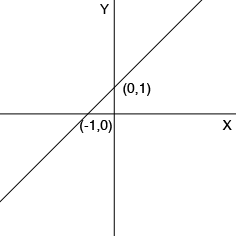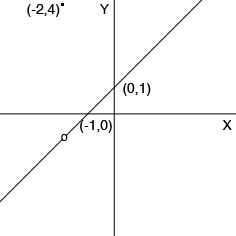SEARCH HOMEMath Central Quandaries & QueriesQuestion from uday, a student: f(x)={x^2+3x+2 / x+2, x(not equal to)=-1 {4, x=-1 how to find domain and how the graph looks likeHi,

I am going to change the function slightly as I think you have copied it incorrectly. I want the expression to be

$f(x) = \left\{ \begin{array}{ll}\frac{x^2 + 3x + 2}{x + 2} & \mbox{if x \neq -2}\\4 & \mbox{if x = -2}. \end{array} \right.$

The value of $-2$ is important since the expression

$\frac{x^2 + 3x + 2}{x + 2}$

doesn't exist at $x = -2$ since the denominator is zero. Looking at the expression

$\frac{x^2 + 3x + 2}{x + 2}$

more closely you can see that the numerator factors so that

$\frac{x^2 + 3x + 2}{x + 2} = \frac{(x + 2)(x + 1)}{x + 2}.$

Thus using cancellation it seems that the expressions $\large \frac{x^2 + 3x + 2}{x + 2}$ and $x + 1$ are equal but that is not true since the first expression doesn't exist for $x = -2$ and the second expression has value $-1$ for $x = -2.$

Now back to the problem of the domain and graph of

$f(x) = \left( \begin{array}{ll}\frac{x^2 + 3x + 2}{x + 2} & \mbox{if x \neq -2}\\4 & \mbox{if x = -2}. \end{array} \right.$

If $x \neq -2$ then $f(x)$ is evaluated using $\large \frac{x^2 + 3x + 2}{x + 2}$ and for $x = -2, \; f(x) = f(-2) = 4$ and thus $f(x)$ exists for all real numbers $x$ and thus the domain of $f(x)$ is all real numbers.

To graph $y = f(x)$ I would start by graphing $y = x + 1$ which is the line with slope 1 and passing through $(0, 1).$y = x + 1

We know that the functions $y = x + 1$ and $y = f(x)$ have the same values for all $x$ except $x = -2.$ At $x = -2$ we have $f(x) = f(-2) = 4$ and thus my graph of $y = f(x) isy = f(x) The small circle on the line at$x = -2$is to indicate that there is a "hole" in the line there and the value of$f(-2)\$ is 4.

I hope this helps,
PennyMath Central is supported by the University of Regina and the Imperial Oil Foundation.